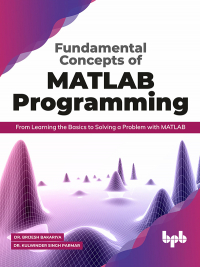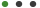# Fundamental Concepts of MATLAB Programming

Formats - EPUB, PDF

Pages - 316

ISBN - 9789389845822#### Description

Learn how to use MATLAB commands and functions in an efficient and effective manner

## Key Features

â— Get familiar and work with the in-built functions in MATLAB
â— Learn how to solve algebraic equations in MATLAB
â— Explore various techniques for plotting numerical data
â— Learn how to preprocess data to ensure accurate, efficient, and meaningful analysis
â— Learn how to issue commands to create variables and call functions

## Description

MATLAB has been an essential platform for data computation. There are various types of technologies that are going on, but it requires a tool for data handling. MATLAB provides better computing power for a massive amount of data.

This book will be your comprehensive guide to creating applications, simulation, computation measures. The book begins with an introduction MATLAB and quickly goes on to teach you the usage of MATLAB. After this, we will explore the various commands and essential concepts and topics about MATLAB. Moving forward, we'll explore importing and exporting data, handling data, and visualization of data through different ways to plot a graph. Towards the end, we will explore the basic algebraic functions used in MATLAB.

## What will you learn

â— Learn how to build and run MATLAB statements
â— Execute a block of code repeatedly using the Loop Control Statements
â— Create a user-defined function by using MATLAB
â— Create, Concatenate, and Expand the most basic MATLAB data structure; Matrix
â— Understand how to plot a 2D and 3D graph

## Who this book is for

This book is for everyone from the Engineering and Sciences background. It is also for PGDCA, B.Tech. B.E., BCA, BSc, M.Tech. /M.E., MCA, M.Com., MSc, Ph.D. other UG, and PG degree students.

1. Basics of MATLAB
2. Expressions and Basic Commands of MATLAB
3. Data Types, Variables and Operators
4. Decision Control Statements
5. Loops Control Statements
6. Vectors
7. Matrix
8. Arrays
9. Strings
10. Functions
11. Data Import and Export
12. Plotting a Graph
13. Graphics
14. Basic Algebra in MATLAB

No Datials Available